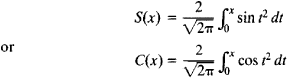Fresnel Integral

Also found in: Wikipedia.
The following article is from The Great Soviet Encyclopedia (1979). It might be outdated or ideologically biased.

Fresnel Integral

Integrals of the formare called Fresnel integrals. They were introduced by A. J. Fresnel for the solution of problems of the diffraction of light. In the case of improper Fresnel integrals, we have S(∞) = C(∞) = ½. Tables of Fresnel integrals are given in many handbooks (for example, E. Jahnke, F. Emde, and F. Lösch, Spetsial’nye funktsii, 2nd ed., 1968 [translated from German]).

Mentioned in ?
References in periodicals archive ?
The Fresnel integral [F.sub.I][F([sigma])] is defined for F([sigma]) [member of] F(H) by the formula
The choice [PHI] (x) = (i/2h)[[absolute value of (x)].sup.2] is of particular interest and is known under the name of Fresnel integral. This choice gives us a mean, up to normalization by a factor [(2i[pi]h).sup.-d/2], and can be generalized to a Hilbert space H the following way.
In this case, it is called infinite dimensional Fresnel integral of f and noted by
The Cartesian coordinates (X, Y) of the transition curves are calculated via using Fresnel integral (Jeffrey, Dai 2008).
These integrals are known as the Fresnel Integral (Jeffrey, Dai 2008).
In either case, the diffraction pattern due to the principal aperture A can be evaluated using a generalized Fresnel integral derived by this author from the general equations for the propagation of cross-spectral density in a partially coherent optical field (3).
Formula (5) and (6) only existing numerical solution, and when the integral upper limit tends to infinity, the two Fresnel integrals all tend to [square root of (2[pi]/4)], i.e.
The necessary integrals can be expressed in terms of Fresnel integrals, and he goes on to explain how to evaluate these special functions.
He pays special attention to formulas of derivatives of nth-order (with respect to the argument) and of the first derivatives (with respect to the parameter) for most elementary and special functions, covering the derivatives (including the Hurwitz zeta function and Fresnel integrals) limits (including special functions), indefinite integrals (including elementary and special functions), definite integrals (including Bessel, Mcdonald, Struve, Kelvin, Legendre, Chebyshev, Hermite, Laguerre and Jacobi functions and polynomials), finite sums, infinite series, the connection formulas and representations of hypergeometric functions and of the Meijer G function.
In the Fresnel limit on either side of the aperture plane (|z| [much greater than] [lambda]) the forward and reverse fields are unidirectional, and the forward field is reduced to the standard expressions in terms of Fresnel integrals for slits and Lommel functions for circular apertures  for z > 0, and to the unperturbed geometrical field for z < 0.
While this integral has an analytic form in terms of the Fresnel integrals, a simple approximation is more useful in this context.
Error Functions, Dawson's Integral, Fresnel Integrals

Site: Follow: Share:
Open / Close